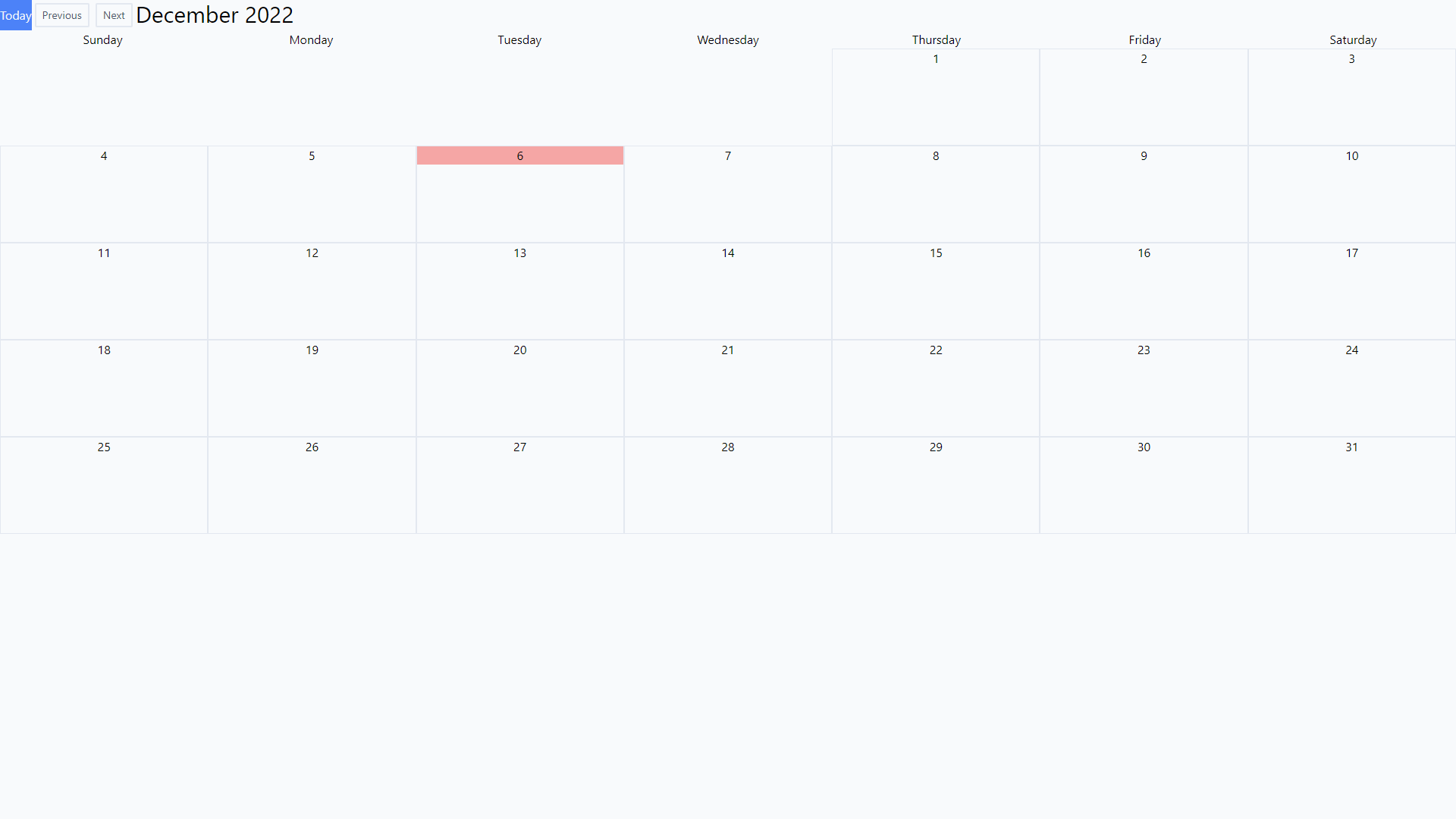# Build Calendar view with vue3 and tailwind

As JS libraries and frameworks keeps churning, I find myself looking for libraries that are actively maintained. UI libraries tend to be forgotten and end up unmaintained or worse they break when a large update is published. That's why, when I can, I build my own custom components and keep it as simple as humanly possible. Calendar components are a good example of a lib I struggle to find one that works. Every project has its own requirements and using an out of the box UI rarely works.

With CSS grid building your own is now fairly easy so the following is a quick skeleton calendar, customizable to fit your needs.To get started you'll need

• Vue3
• Tailwind
• dayjs

``<template></template><script setup lang='ts'></script>``

Let's define some properties. We'll need a modelValue prop to feed in events and a startDate that sets the initial month to display.

``<script setup lang='ts'>type Props = {    modelValue?: any    startDate?: string    }const props = withDefaults(defineProps<Props>(), {    modelValue: () => null,        startDate: () => '2022-12-05'});const emits = defineEmits(['update:modelValue']);</script>``

Next comes a function to calculates the day in a given month. Dayjs has all the functions to work that out, so let's import.
Adding the function as a computed property will make it reactive. Every time viewDate changes, the range is recalculated.

``<script setup lang='ts'>import { computed, ref } from 'vue';import dayjs from 'dayjs';const viewDate = ref(dayjs(props.startDate));const units = computed(() => {    let ranges = [];    let startOfRange = viewDate.value.startOf('month').add(-1,'day');    let endOfRange = viewDate.value.endOf('month').add(-1,'day');    let currentDate = startOfRange;    while (currentDate.isBefore(endOfRange) || currentDate.isSame(endOfRange)) {        currentDate = currentDate.add(1, 'day');        ranges.push(currentDate);    }    return ranges;})</script>``

Now Let's add a css grid to show our calendar boxes

``<template>      <div class="grid grid-cols-7">                <div class="border border-slate-200 flex flex-col h-32"             v-for="d in units">            <div class="text-center">{{ d.format('D') }}</div>              </div>    </div></template>``

Most calendars, show the days of the week at the very top. To make that work, we'll need to align the 1st day of the month with the correct day. So we'll need a second function to calculate that gap

``const daystoPrepend = computed(() => {    const startOfMonth = viewDate.value.startOf("month");    const startOfFirstWeek = startOfMonth.startOf("week");    const daysToFirstDay = startOfMonth.diff(startOfFirstWeek, "day");    return Array.from(new Array(daysToFirstDay).keys());})``

and let's add empty boxes in front to align our days.

``    <div class="grid grid-cols-7">        <div v-for="p in daystoPrepend"></div>        <div class="border border-slate-200 flex flex-col h-32"             v-for="d in units">            <div class="text-center">{{ d.format('D') }}</div>                   </div>    </div>``

Now we can add the days on top of the calendar.

``<template>    <div class="grid grid-cols-7 gap-1">        <div v-for="d in weekDays"             class="text-center">            <div>{{ d }}</div>        </div>    </div>    <div class="grid grid-cols-7">        <div v-for="p in daystoPrepend"></div>        <div class="border border-slate-200 flex flex-col h-32"             v-for="d in units">            <div class="text-center">{{ d.format('D') }}</div>              </div>    </div></template>const weekDays = [    'Sunday',    'Monday',    'Tuesday',    'Wednesday',    'Thursday',    'Friday',    'Saturday',]</script>``

Finally we'll need some buttons to navigate between months and reset to today. Since everything is computed from viewDate we just need to manipulate that value.

``<div class="flex">    <button class="btn-primary"            @click="reset()">Today</button>    <button class="btn"            @click="shiftMonth(-1)">Previous</button>    <button class="btn"            @click="shiftMonth(1)">Next</button>    <span class="text-3xl">{{ viewDate.format('MMMM YYYY') }}</span></div>const shiftMonth = function (amount: number) {    viewDate.value = viewDate.value.add(amount, 'month');}const reset = function () {    viewDate.value = dayjs();}</script>``

and we're done 😊 pass your content in modelValue, render them and 💰

Here is the full code for reference.

``<template>    <div class="flex">        <button class="btn-primary"                @click="reset()">Today</button>        <button class="btn"                @click="shiftMonth(-1)">Previous</button>        <button class="btn"                @click="shiftMonth(1)">Next</button>        <span class="text-3xl">{{ viewDate.format('MMMM YYYY') }}</span>    </div>    <div class="grid grid-cols-7 gap-1">        <div v-for="d in weekDays"             class="text-center">            <div>{{ d }}</div>        </div>    </div>    <div class="grid grid-cols-7">        <div v-for="p in daystoPrepend"></div>        <div class="border border-slate-200 flex flex-col h-32"             v-for="d in units">            <div :class="[d.isToday() ? 'bg-red-300' : '']" class="text-center">{{ d.format('D') }}</div>                    </div>    </div></template><script setup lang='ts'>import { ISODate } from '\$/common/types/Notebook';import { computed, ref } from 'vue';import dayjs from 'dayjs';import isToday from 'dayjs/plugin/isToday'dayjs.extend(isToday);type Props = {    modelValue?: any    startDate?: ISODate    display?: 'month' | 'year' | 'week' | 'day'}const props = withDefaults(defineProps<Props>(), {    modelValue: () => null,    display: () => 'month',    startDate: () => '2022-12-05'});const emits = defineEmits(['update:modelValue']);const viewDate = ref(dayjs(props.startDate));const daystoPrepend = computed(() => {    const startOfMonth = viewDate.value.startOf("month");    const startOfFirstWeek = startOfMonth.startOf("week");    const daysToFirstDay = startOfMonth.diff(startOfFirstWeek, "day");    return Array.from(new Array(daysToFirstDay).keys());})const units = computed(() => {    let ranges = [];    let startOfRange = viewDate.value.startOf('month').add(-1,'day');    let endOfRange = viewDate.value.endOf('month').add(-1,'day');    let currentDate = startOfRange;    while (currentDate.isBefore(endOfRange) || currentDate.isSame(endOfRange)) {        currentDate = currentDate.add(1, 'day');        ranges.push(currentDate);    }    return ranges;})const shiftMonth = function (amount: number) {    viewDate.value = viewDate.value.add(amount, 'month');}const reset = function () {    viewDate.value = dayjs();}const weekDays = [    'Sunday',    'Monday',    'Tuesday',    'Wednesday',    'Thursday',    'Friday',    'Saturday',]</script>``